# Simulating leveraged etfs¶

A while ago I have written a post about leveraged etfs. While many people think that leveraged etfs decay over time, this is actually not the case. What we see is in fact a non-symmetric distribution, with a high probability of decay, but with the same expected per timestep as the non-leveraged distribution.

To prove this, we can use Monte-Carlo simulation (which is a fancy name for a brute-force approach) to test this hypothesis.

Before we start, there is one thing that needs a little bit of explanation: libraries

### Import modules¶

Python has only a limited set of built-in functions. Everyhing else needs to be loaded from optional modules (Don't get confused, modules and libraries are the same thing). We will use %pylab magic to load most of the scientific libraries, including plotting functions.

We will be looking at modules later on, for now it is enough just to start the notebook and run the %pylab inline command. inline causes the figures to appear inside the notebok instead of a popup window.

In :
%pylab inline

Populating the interactive namespace from numpy and matplotlib

In :
%run common.py


### Create a synthetic price¶

Suppose we have an etf with normally distributed daily returns. Average daily return is 0 and standard deviation is 1%. In other words chances of going up 1% are equal to chances of going down 1%

In :
returns = randn(10000) # create a 1000-day returns vector.
#Note: don't use 'return' as a variable name, this keyword is reserved.
plot(returns) #plot the time series
grid(True) #use grid in the plot

title('Daily returns')
xlabel('day')
ylabel('return [%]')

Out:
<matplotlib.text.Text at 0x7f0b70e9b2e8>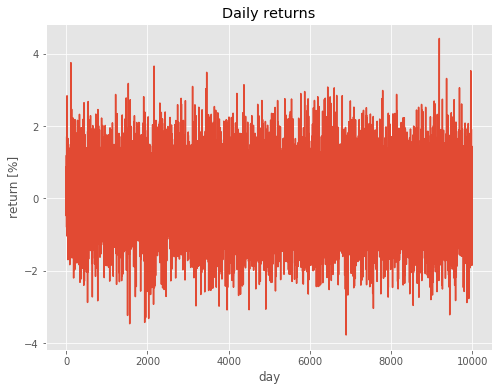Let's see how this distribution looks on a histogram and check if mean (average) and std (standard deviation) are what we expect Note: for printing std I am using here string formatting in the form of %.2f, which means that a floating number is printed with two decimals precision.

In :
h = hist(returns,100) # make a histogram of returns, using 100 bins.
#h is used as a return variable to avoid text output

print('Mean:' , returns.mean()) # print mean
print('Standard deviation:%.2f' % returns.std()) # print sigma, note the 2 decimal precision notation

Mean: 0.012487743073
Standard deviation:0.99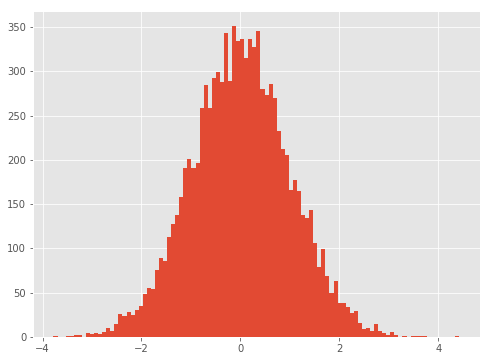Suppose we would start on day 0 with an investment of $100. On day n our investment would then have a value of 100*r[t]*r[t+1]*...r[n], which is achieved by the cumulative product function. Don't forget to divide % returns by 100 and add 1 to the daily returns as we are multiplying by 1.05 in case of 5% return In : price = 100*(returns/100+1).cumprod() # normalized price plot(price) # plot cumulative sum of returns title('normalized price') # set plot title xlabel('day number') # add x label to the chart ylabel('price [$]') # add y label

Out:
<matplotlib.text.Text at 0x7f0b6eb50da0>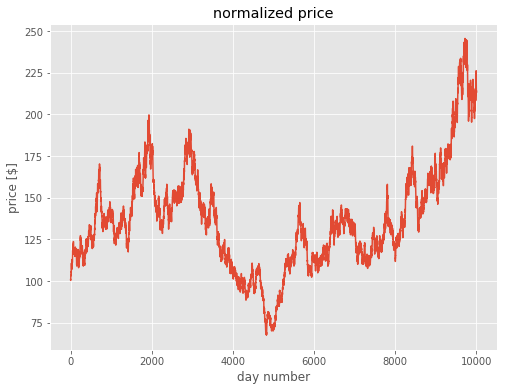You will notice that each time you run the code in the previous cells, the result is different. This is of course because we used the random number generator. Now we can do the same calculation, but for many simulated prices at once. We could use a loop for this and repeat the above calculation many times, but there is a more elegant way. (note: the returns variable from previous calculations is overwritten with a matrix)

In :
returns = randn(1000,5000) # generate 1000x5000 random return series
prices = 100*(returns/100+1).cumprod(axis=0) # normalized price, you need to specify along which axis
#(0 is along columns) the cumulative product is calculated.
h = plot(prices[:,:500]) # plot cumulative sum of returns. Because plotting all 5000 prices would take
# a long time, only first 500 are plotted
xlabel('day number')
ylabel('price [$]')  Out: <matplotlib.text.Text at 0x7f0b6eb96ba8>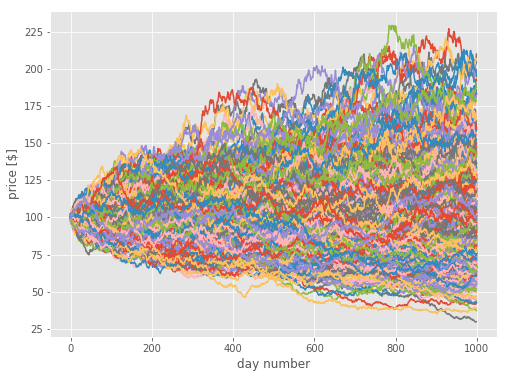The final price (on the 1000th day) of the prices matrix (or table if you like spreadsheet terminology) is just the last row. Let's plot a histogram of it. In : finalPrice = prices[-1,:] # get the last row of prices table h = hist(finalPrice,50) # plot histogram with 50 bins xlabel('price [$]') # add axis labels
ylabel('frequency [#]')

print('Mean:', finalPrice.mean()) # show mean value
print('Std:', finalPrice.std()) # show standard deviation

Mean: 99.9924210971
Std: 31.9031817194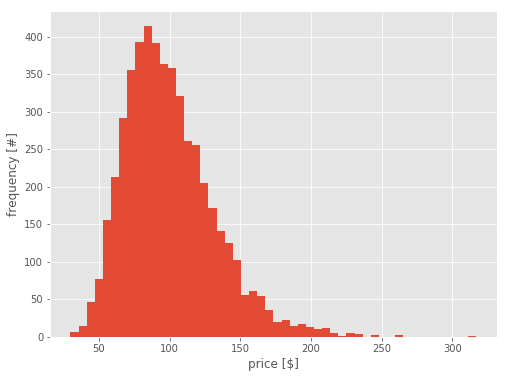The mean of resulting distribution is very close to our starting value of 100$, but the distribution is now skewed to the right. Calculating standard deviation for this distribution can be misleading, because it is not a normal distribution any more. conclusion : an equal chance of going up or down in % does not change long term expected value. The resulting distribution is however skewed, therefore we see a price decline more often, but it is compensated by the fat tails on the up side. ## How about 3x leverage?¶ Let's calculate and plot a 3x leveraged result. We can reuse the return matrix from previous calculation In : returns3x = returns*3. # leveraged returns are 3x the non-leveraged returns prices3x = 100*(returns3x/100+1).cumprod(axis=0) # normalized price, you need to specify along which axis finalPrice3x = prices3x[-1,:] # get the last row of prices table h1 = hist(finalPrice3x,500) # plot histogram with 500 bins. more bins are needed to keep the bin width small. h2 = hist(finalPrice,100) # plot histogram with 100 bins xlim([0,200]) # set x axis limits to 0..200 legend(['3x leverage','1x leverage']) print('Mean 3x:', finalPrice3x.mean())  Mean 3x: 99.0548961218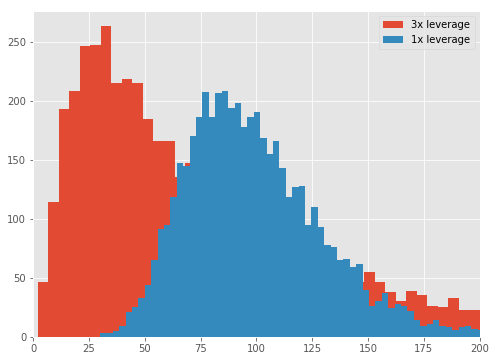That is funny: while the 3x leveraged etf most of the time finishes under the $50 mark, the fat tail on the right keeps the long term average at \$100 The tail on the right is in fact so large, that I had to limit the x-axis.

### Conclusion¶

Long term expected value does not change with increasing etf leverage, but the likelyhood of ending up with a loss increases.

### Excersize¶

The above calculation is done for an etf that has no alpha bias (average daily return is 0%). Most of the stocks however do have a positive bias. Investigate what would happen with if an etf would be based on a normal distribution with an average daily return being 0.001%